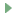## 创建科学项目

### 使用预定义的科学设置创建 PyCharm 项目

1. 打开新建项目向导（文件 | 新建 | 项目）。

2. 选择科学项目类型。

3. 在项目设置对话框窗口中，指定项目名称，确保选择 Conda 作为新环境，并更改默认数据文件夹（如果需要），然后单击创建

4. 打开main.py文件并添加以下代码示例：

将 numpy 作为 np 导入 matplotlib.pyplot 作为 plt N = 50 x = np.random.rand(N) y = np.random.rand(N) 颜色 = np.random.rand(N) 面积 = np.pi * ( 15 * np.random.rand(N))**2 # 0 到 15 点半径 plt.scatter(x, y, s=area, c=colors, alpha=0.5) plt.show() X = np.linspace (-np.pi, np.pi, 256,endpoint=True) C,S = np.cos(X), np.sin(X) plt.plot(X, C, color="blue", linewidth=2.5 , linestyle="-") plt.plot(X, S, color="red", linewidth=2.5, linestyle="-") plt.xlim(X.min()*1.1, X.max()*1.1 ) plt.xticks([-np.pi, -np.pi/2, 0, np.pi/2, np.pi], [r'$-\pi$', r'$-\pi/2$', r'$0$', r'$+\pi/2$', r'$+\pi$']) plt.ylim(C.min()*1.1,C. max()*1.1) plt.yticks([-1, 0, +1], [r'$-1$', r'$0$', r'$+1$']) plt.show()
5. numpy处理为和导入显示的警告matplotlib并启用项目中的包。

## 跑步

### 要执行代码单元：

1. 通过添加“#%%”行来修改main.py文件。

2. 在装订Ctrl+Enter线中，单击与散点图单元格标记对齐的图标。只会构建散点图。

3. 现在单击图标或按Ctrl+Enter带有 y 对 x 绘图单元标记的行。应出现相应的图表。

plt.show()

## 概括

• 文件main.py已创建并打开以进行编辑。

• 源码已经输入（注意PyCharm强大的代码补全功能！）

• 源代码已经运行和调试。

• 最后，我们在控制台中运行该文件。